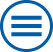## The group contributes to the following mathematical research topics of WIAS:

Analysis of Partial Differential Equations and Evolutionary Equations

Partial differential equations form an adequate and powerful instrument to provide a mathematical model for nature. At the Weierstrass Institute this research has three essential focuses: (a) Rigorous mathematical analysis of general evolution equations in terms of existence, uniqueness and regularity of different types of solutions, (b) Development of variational approaches using the toolbox of the calculus of variations, (c) Regularity results for solutions of elliptic and parabolic partial differential equations. [>> more]

Free boundary problems for partial differential equations

Free boundary problems are investigated in connection with energy technology and coating of surfaces. [>> more]

Functional analysis and operator theory

At WIAS, functional analysis and operator theory are related, in particular, to problems of partial differential equations and evolutions equations, to analysis of multiscale, hybrid and rate-independent models. [>> more]

Numerical methods for coupled systems in computational fluid dynamics

The main field of research are schemes for convection-diffusion equations, transport equations with exponential nonlinearities and Navier-Stokes equations (turbulent flows). The methods are based on FEM and FVM spatial discretizations and on implicit temporal discretizations. Considered applications involve population balance systems and Roosbroeck systems. [>> more]

Optimal control of partial differential equations and nonlinear optimization

Many processes in nature and technics can only be prescribed by partial differential equations,e.g. heating- or cooling processes, the propagation of acoustic or electromagnetic waves, fluid mechanics. Additionally to challenges in modeling, in various applications the manipulation or controlling of the modeled system is also of interest in order to obtain a certain purpose... [>> more]

Stochastic Optimization

Stochastic Optimization in the widest sense is concerned with optimization problems influenced by random parameters in the objective or constraints. [>> more]

Systems of partial differential equations: modeling, numerical analysis and simulation

The mathematical description of many scientific and technological problems leads to systems of partial differential equations (PDEs). [>> more]

Variational methods

Many physical phenomena can be described by suitable functionals, whose critical points play the role of equilibrium solutions. Of particular interest are local and global minimizers: a soap bubble minimizes the surface area subject to a given volume and an elastic body minimizes the stored elastic energy subject to given boundary conditions. [>> more]